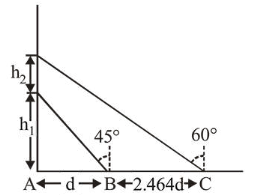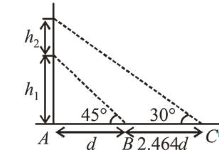# A balloon is moving up in air vertically above a point

Question:

A balloon is moving up in air vertically above a point $\mathrm{A}$ on the ground. When it is at a height $h_{1}$, a girl standing at a distance $d$ (point B) from A (see figure) sees it at an angle $45^{\circ}$ with respect to the vertical. When the balloon climbs up a further height $h_{2}$, it is seen at an angle $60^{\circ}$ with respect to the vertical if the girl moves further by a distance $2.464 d$ (point C). Then the height $h_{2}$ is (given $\tan 30^{\circ}=$ $0.5774)$ :1. (1) $1.464 d$

2. (2) $0.732 d$c

3. (3) $0.464 d$

4. (4) $d$

Correct Option: , 4

Solution:

(4) From figure/ trigonometry,

$\frac{h_{1}}{d}=\tan 45^{\circ}$'                    $\therefore h_{1}=d$And, $\frac{h_{1}+h_{2}}{d+2.464 d}=\tan 30^{\circ}$

$\Rightarrow\left(h_{1}+h_{2}\right) \times \sqrt{3}=3.46 d$

$\Rightarrow\left(h_{1}+h_{2}\right)=\frac{3.46 d}{\sqrt{3}} \Rightarrow d+h_{2}=\frac{3.46 d}{\sqrt{3}}$

$\therefore h_{2}=d$